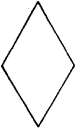# rhombus

(redirected from rhombuses)
Also found in: Dictionary, Thesaurus.
Related to rhombuses: rhombi, parallelogram

## rhombus

an oblique-angled parallelogram having four equal sides
Collins Discovery Encyclopedia, 1st edition © HarperCollins Publishers 2005
The following article is from The Great Soviet Encyclopedia (1979). It might be outdated or ideologically biased.

## Rhombus

a plane quadrangle with equal sides (Figure 1). It may be regarded as the special case of the parallelogram where two adjacent sides are equal, the diagonals are perpendicular,Figure 1

or a diagonal bisects the angles of the vertices that it joins. A rhombus with right angles is called a square.

## rhombus

[′räm·bəs]
(mathematics)
A parallelogram with all sides equal. Also known as rhomb.
McGraw-Hill Dictionary of Scientific & Technical Terms, 6E, Copyright © 2003 by The McGraw-Hill Companies, Inc.
References in periodicals archive ?
Things are less trivial for rhombuses, since the definition of the open-shell manifold is ambiguous.
Due to restrictions of the MOLPRO package all the calculations were done using abelian point groups but imposing [D.sub.6h], [D.sub.2h], and [D.sub.3h] symmetries on the hexagons, rhombuses, and triangles coordinates, respectively.
As Penrose patterns can be built up out of two different types of rhombuses, so the spatial versions of the Penrose patterns can be constructed from different rhombic polyhedrons or rhombohedrons.
If the letters of the L edge word read upwards 54321 (see Rhombuses above), the transposed letters of the R edge word conform to one of 5 permutations (both the last letter and the initial letter being predetermined).
With 5 letters, the edge words of rhombuses cannot be tautonyms.
Distribute paper cutouts to groups; each group should get at least three different sizes of one type of shape (i.e., three different-sized rectangles, rhombuses, or parallellograms), and each person in the group should get his or her own cutout with which to work.
Then have the groups that worked with rhombuses report their results.
Others may be able to use the relationships between rhombuses and triangles to figure out how many triangles cover the shape.
They then fill the outline with the fewest blocks possible and record their results (seven blue rhombuses would be used in the problem shown in fig.
[ILLUSTRATION OMITTED] How many rhombuses like this [ILLUSTRATION OMITTED] does it take to cover the hexagon?
Since it took three to cover one of these [placing two rhombuses in the upper-left hexagon position], that would be three [pointing to the two leftmost hexagons in the shape], six [see fig.

Site: Follow: Share:
Open / Close